# 一、何为决策树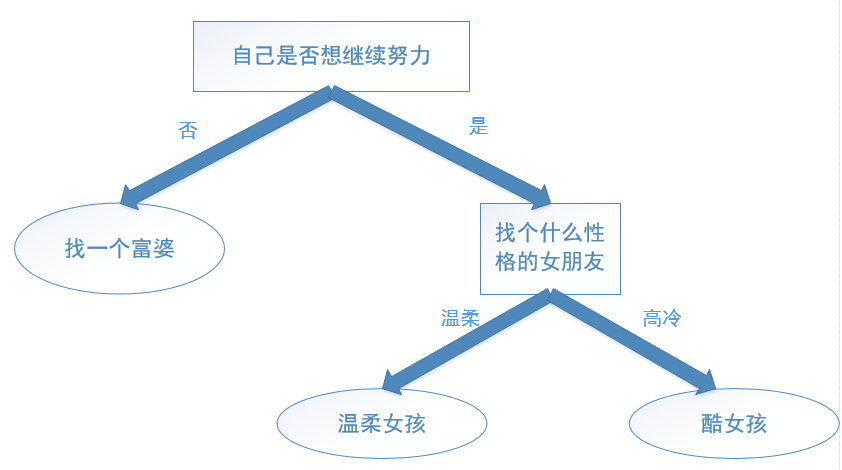1. 根节点到每一个子节点的路径可构成一条规则。
2. 每条路径上中间节点的特征对应该条规则的判断条件，叶子节点的标签对应该规则的结论。
3. 每一个实例都被有且仅有一条实例覆盖，即实例的特征与路径上的特征一致。

# 二、决策树的流程

4. 收集数据:公开数据源或爬虫等方式。
5. 准备数据:树构造算法只适用于标称型数据，因此数值型数据必须离散化。
6. 分析数据:可以使用任何方法，构造树完成之后，需检查图形是否符合预期。
7. 训练算法:构造树的数据结构。
8. 测试算法:计算树模型的正确率。
9. 使用算法:此步骤可以适用于任何监督学习算法，决策树可视化能更好地理解数据的内在含义。

# 三、特征选择

no surfacing flippers fish

### 3.1 熵(香农熵)### 3.2计算熵

``````#创建数据集
def createDataSet():
DataSet =  [[1, 1, 'yes'],
[1, 1, 'yes'],
[1, 0, 'no'],
[0, 1, 'no'],
[0, 1, 'no']]
#矩阵转化Dataframe
DataSet = pd.DataFrame(DataSet,columns=['no surfacing','flippers','fish'])
return DataSet
#计算信息熵
def calculatent(DataSet):
#统计类标签的分类
fish_type = DataSet['fish'].value_counts()
'''
no     3
yes    2
'''
#获取样本个数
m = DataSet.shape
#每个分类的概率，即p(Xi)
p = fish_type/m
#计算熵值
ent = (-p*np.log2(p)).sum()
return ent
'''
0.9709505944546686
'''``````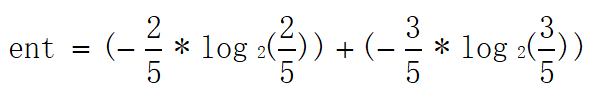### 3.3信息增益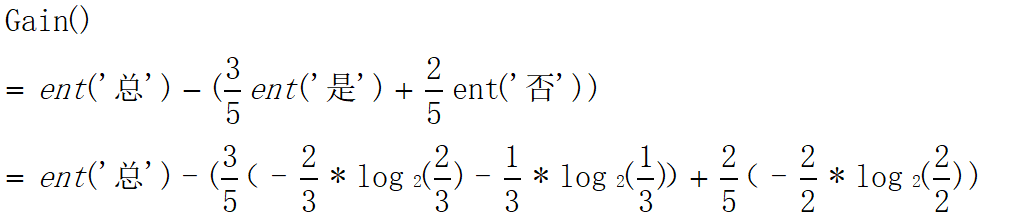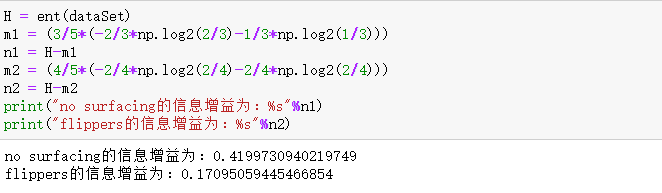``````#最优特征函数
def ChooseBF(DataSet):
#获取基础信息熵
all_ent = calculatent(DataSet)
BestGain = 0 #初始化一个信息增益
col = -1 #初始化一个最优特征索引
for i in range(DataSet.shape-1): #遍历所有特征
#获取第i列的分类
index_ = DataSet.iloc[:,i].value_counts().index
child_ent = 0 #初始化某列的信息熵
for j in index_:
#将第i列按照类别分类
child_DataSet = DataSet[DataSet.iloc[:,i]==j]
#计算某一类别的信息熵
ent = calculatent(child_DataSet)
#将所有类别信息熵加权求和
child_ent += (child_DataSet.shape/DataSet.shape)*ent
print("第%s列的熵为%s"%(i,child_ent))
TheGain = all_ent-child_ent #获取信息增益
print("第%s列的信息增益为%s"%(i,TheGain))
#得出最优特征索引
if(TheGain>BestGain):
BestGain = TheGain
col = i
print("最优特征索引为：%s"%col)
return col``````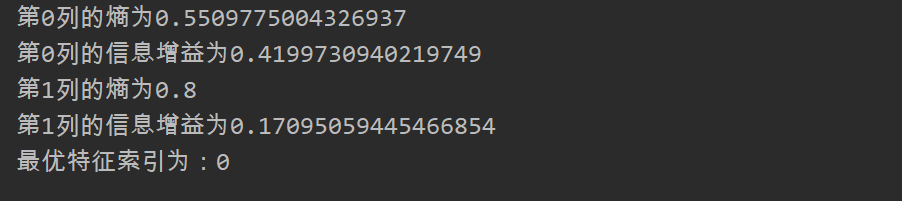``````#切分数据集函数
def splitSet(DataSet,col,value):
#找到最优特征索引标签
index = (DataSet.iloc[:,col]).name
#切分数据集，并把当前最优特征删去
resetdata = DataSet[DataSet.iloc[:,col]==value].drop(index,axis = 1)
return resetdata``````

``````    '''
col = 0,value = 1
flippers fish
0         1  yes
1         1  yes
2         0   no
col = 0,value = 0
flippers fish
3         1   no
4         1   no
'''``````